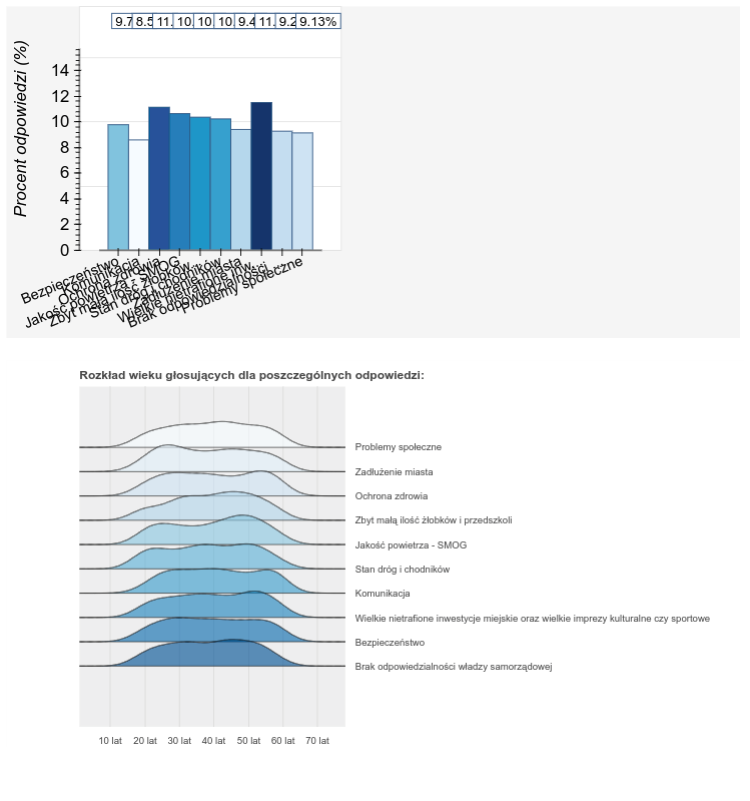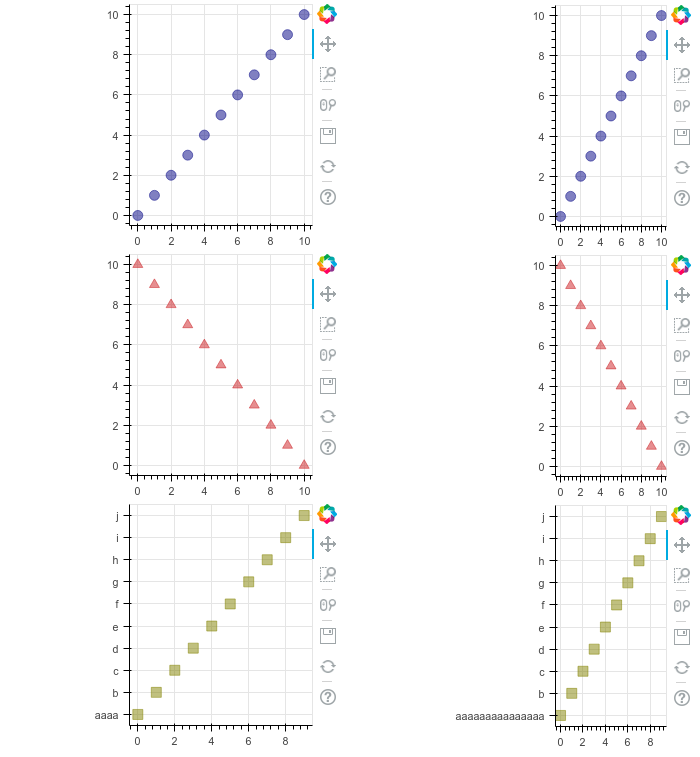# How to decouple the width of plots being in the same layout column?

Hello there,

Is there a way to set widths of the plots separately within the same column?

I have an issue which is well visible on the plot below. The categorical axis labels on the bottom plot are causing shrinking of the top plot as well. I would like to have top plot span whole available width of the page.Such behavior is easily reproducible using such a minimal example:

from bokeh.io import output_file, show
from bokeh.layouts import column
from bokeh.plotting import figure

output_file(“layout.html”)

x = list(range(11))
y0 = x
y1 = [10 - i for i in x]
y2 = [abs(i - 5) for i in x]

keys = [“aaaaaaaaaaaaaaa”,
“b”,
“c”,
“d”,
“e”,
“f”,
“g”,
“h”,
“i”,
“j”]

# create a new plot

s1 = figure(plot_width=250, plot_height=250, title=None)
s1.circle(x, y0, size=10, color=“navy”, alpha=0.5)

# create another one

s2 = figure(plot_width=250, plot_height=250, title=None)
s2.triangle(x, y1, size=10, color=“firebrick”, alpha=0.5)

# create and another

s3 = figure(plot_width=250, plot_height=250, title=None, y_range=keys)
s3.square(x, keys, size=10, color=“olive”, alpha=0.5)

# put the results in a column and show

show(column(s1, s2, s3))

``

And again for the two top plots there is whole lot of unwanted blank space on the left (as the labels are getting longer):Is there any workaround? Or maybe there is some specific sizing_mode, that I should use with my layout?

Cheers,

Maciej Lewicki

Hi,

Since this is a standalone (i.e. not server) document, I would recommend using "components" to embed the plots separately in your own HTML template:

Then if you want one plot to have scale_width sizing, and another fixed sizing, that will be possible.

Thanks,

Bryan

···

On Mar 27, 2018, at 08:49, [email protected] wrote:

Hello there,

Is there a way to set widths of the plots separately within the same column?
I have an issue which is well visible on the plot below. The categorical axis labels on the bottom plot are causing shrinking of the top plot as well. I would like to have top plot span whole available width of the page.

Such behavior is easily reproducible using such a minimal example:

from bokeh.io import output_file, show
from bokeh.layouts import column
from bokeh.plotting import figure

output_file("layout.html")

x = list(range(11))
y0 = x
y1 = [10 - i for i in x]
y2 = [abs(i - 5) for i in x]

keys = ["aaaaaaaaaaaaaaa",
"b",
"c",
"d",
"e",
"f",
"g",
"h",
"i",
"j"]

# create a new plot
s1 = figure(plot_width=250, plot_height=250, title=None)
s1.circle(x, y0, size=10, color="navy", alpha=0.5)

# create another one
s2 = figure(plot_width=250, plot_height=250, title=None)
s2.triangle(x, y1, size=10, color="firebrick", alpha=0.5)

# create and another
s3 = figure(plot_width=250, plot_height=250, title=None, y_range=keys)
s3.square(x, keys, size=10, color="olive", alpha=0.5)

# put the results in a column and show
show(column(s1, s2, s3))

And again for the two top plots there is whole lot of unwanted blank space on the left (as the labels are getting longer):

Is there any workaround? Or maybe there is some specific sizing_mode, that I should use with my layout?

Cheers,

Maciej Lewicki

--
You received this message because you are subscribed to the Google Groups "Bokeh Discussion - Public" group.
To unsubscribe from this group and stop receiving emails from it, send an email to [email protected].
To post to this group, send email to [email protected].
To view this discussion on the web visit https://groups.google.com/a/continuum.io/d/msgid/bokeh/3c6a56c9-461f-49d8-85bc-781e7e53db41%40continuum.io.

Hi,

How would I go about it in case of a server application? My app (first pic) runs on bokeh server.

Cheers,

Maciek

W dniu wtorek, 27 marca 2018 17:54:12 UTC+2 użytkownik Bryan Van de ven napisał:

···

Hi,

Since this is a standalone (i.e. not server) document, I would recommend using “components” to embed the plots separately in your own HTML template:

``````    [https://bokeh.pydata.org/en/latest/docs/user_guide/embed.html#components](https://bokeh.pydata.org/en/latest/docs/user_guide/embed.html#components)
``````

Then if you want one plot to have scale_width sizing, and another fixed sizing, that will be possible.

Thanks,

Bryan

On Mar 27, 2018, at 08:49, [email protected] wrote:

Hello there,

Is there a way to set widths of the plots separately within the same column?

I have an issue which is well visible on the plot below. The categorical axis labels on the bottom plot are causing shrinking of the top plot as well. I would like to have top plot span whole available width of the page.

Such behavior is easily reproducible using such a minimal example:

from bokeh.io import output_file, show

from bokeh.layouts import column

from bokeh.plotting import figure

output_file(“layout.html”)

x = list(range(11))

y0 = x

y1 = [10 - i for i in x]

y2 = [abs(i - 5) for i in x]

keys = [“aaaaaaaaaaaaaaa”,

``````    "b",
``````
``````    "c",
``````
``````    "d",
``````
``````    "e",
``````
``````    "f",
``````
``````    "g",
``````
``````    "h",
``````
``````    "i",
``````
``````    "j"]
``````

# create a new plot

s1 = figure(plot_width=250, plot_height=250, title=None)

s1.circle(x, y0, size=10, color=“navy”, alpha=0.5)

# create another one

s2 = figure(plot_width=250, plot_height=250, title=None)

s2.triangle(x, y1, size=10, color=“firebrick”, alpha=0.5)

# create and another

s3 = figure(plot_width=250, plot_height=250, title=None, y_range=keys)

s3.square(x, keys, size=10, color=“olive”, alpha=0.5)

# put the results in a column and show

show(column(s1, s2, s3))

And again for the two top plots there is whole lot of unwanted blank space on the left (as the labels are getting longer):

Is there any workaround? Or maybe there is some specific sizing_mode, that I should use with my layout?

Cheers,

Maciej Lewicki

You received this message because you are subscribed to the Google Groups “Bokeh Discussion - Public” group.

To unsubscribe from this group and stop receiving emails from it, send an email to [email protected].

To post to this group, send email to [email protected].

To view this discussion on the web visit https://groups.google.com/a/continuum.io/d/msgid/bokeh/3c6a56c9-461f-49d8-85bc-781e7e53db41%40continuum.io.